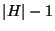Next: Logic Up: Games and Puzzles Previous: MINIMUM GRAPH MOTION PLANNING   Index

### MINIMUM TRAVEL ROBOT LOCALIZATION

• INSTANCE: A simple polygon P and the visibility polygon V of the location of a robot inside P.

• SOLUTION: A motion scheme for the robot to determine its exact location.

• MEASURE: The distance the robot travels according to the motion scheme.

• Good News: Approximable withinwhere H is the set of points in P with visibility polygon V .

• Comment: The problem SHORTEST WATCHMAN ROUTE where the polygon P have holes and the problem is to find a shortest tour inside P such that the robot can see all of P is approximable within, where m is the minimum number of edges in an optimal rectilinear tour .

Viggo Kann
2000-03-20